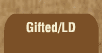Mathematics and Dyslexia

Tips for Learning the Times Tables

Tools for the Times Tables & Workbook

Home > Math > times tables practice > Nines times tables in order

## The Nines Times Tables In Order - first set

The digits of the numbers that you can divide by nine always add up to nine. For 18, 1 + 8 is 9; for 27, 2 + 7 is 9, etc. etc.

1. 9 x 0=
2. 9 x 1=
3. 9 x 2=
4. 9 x 3=
5. 9 x 4=
6. 9 x 5=
7. 9 x 2=
8. 3 x 9=
9. 9 x 4=
10. 5 x 9=
11. 9 x 4=
12. 9 x 3=
13. 2 x 9=
14. 9 x 1=
15. 0 x 9=